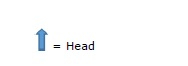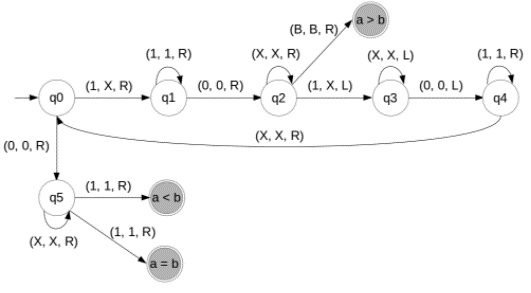# Construct Turing machine for subtraction

A Turing machine is a seven tuples

(Q, Σ,Γ, δ,q0,qacc, qrej)

Where,

• Q is finite number of states
• Σ is the input alphabet does not contain the blank symbol t;
• Γ is the tape alphabet, where t ε Γ and Σ ⊆ Γ;
• δ: (Q × Γ) → (Q × Γ × {L, R}) is the transition function;
• q0 ε Q is the start state;
• qacc ε Q is the accept state;
• qrej ε Q is the reject state, where qrej ≠qacc.

## Problem

Construct a Turing machine (TM) for subtraction of two unary integers.

## Solution

Subtraction of two unary integers

3-2=1

In Turing Machine 3 represents − 111

2 represents: 11

Let ‘M’ be a symbol used to separate two integers

 B 1 1 1 M 1 1 B

Here B= blank

M= Symbol used two separate two integers## Algorithm

Follow the step wise algorithm given below to construct a TM for subtraction −

Step 1 − Let’s take two unary integers as an input. We start from the initial state q0.

Step 2 − If we find the 1 in the string then go to the same state without changing the value of 1, and go to the right of the string.

Step 3 − If we find the m symbol then ignore this symbol without changing the symbol and go to the right of the string.

Step 4 − If we find the 1 after the pass the M symbol then change the value of 1 into the X and go to the left of the string.

Step 5 − Then pass the symbol of M and go to the left and if we find the 1 then change the value of 1 to X and go to the right.

Step 6 − So, this type after the symbol(M) we change all values of 1's into X and the same total number of 1's change into the X before the symbol M.

Step 7 − Apply these steps and get the Turing machine subtraction of two unary integers.

While working with subtraction, the Turing machine considers any of the three cases based on input given by the user.

If ‘a’ and ‘b’ are two integers, we have two consider whether −

• a>b
• a<b
• a=b

By consider all the three conditions, the final Turing machine is as follows−Explanation of the diagram

Step 1 − Consider an input string as 110111

i.e. a=11 and b=111, according to given input a

Step 2 − Scan string from left to right.

Step 3 − Mark '1' as 'X' and then move to the right.

Step 4 − Reaching to the right of '0' and marking '1' as 'X' and moving left.

Step 5 − Reaching 'X' on left of '0' and moving one step right.

Step 6 − Again mark '1' as 'X' and then move to the right.

Step 7 − Reaching to the right of '0' and passing 'X', mark '1' as 'X' and move left.

Step 8 − Reach 'X' in the left of '0'and move one step right.

Step 9 − If there is a ‘0’ after 'X' that means all '1's are finished before '0'.

Step 10 − Pass '0', 'X' and there is '1' remaining. That is, the second number is greater than the first one.

Step 11 − And then the final state is "a<b"